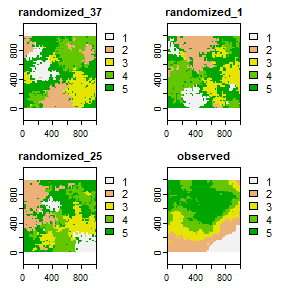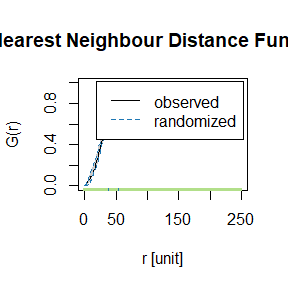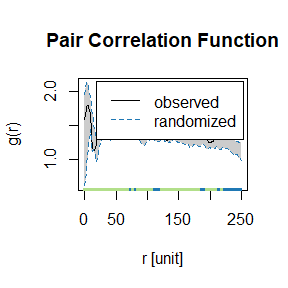# Get started

#### 2023-03-13

library(shar)
library(spatstat)
library(terra)

set.seed(42)

## Design

The core of shar are functions to to simulate null model data by randomizing either the environmental data (i.e. raster data) or the locations of species (i.e. point pattern data). The null data is then used to analyse if significant species-habitat associations are present. Additionally, functions to visualize and analyse the results are available as well as some utility functions. The methods are mainly described in Harms et al. (2001), Plotkin et al. (2000) and Wiegand & Moloney (2014). The methods are not necessary complementary, but are rather different approaches for the same result.

## Preprocessing of input data

All functions are designed for discrete habitat classes. Following, in case only continuous data is available, this has to be classified to discrete classes. classify_habitats provides several ways to classify the data such as the Fisher-Jenks algorithm (Fisher 1958, Jenks & Caspall 1971)

landscape_discrete <- classify_habitats(raster = terra::rast(landscape), n = 5, style = "fisher")

## Randomize environmental data

There are two functions to randomize the environmental data: translate_raster() and randomize_raster(). The first function is a torus translation of the raster, shifting the habitat map in all four cardinal directions. This is only possible for rectangular observation areas and results in n_random <- (terra::nrow(terra::rast(landscape)) + 1) * (terra::ncol(terra::rast(landscape)) + 1) - 4 randomized rasters. The other function randomizes the environmental data using a random-walk algorithm. Here, the number of randomized rasters can be specified using the n_random argument.

However, all methods require “fully mapped data” in a sense that NA cells of the environmental data are allowed only if simultaneously these areas cannot accommodate any locations of the point pattern (e.g., a water body within a forest area). This needs to be reflected in the observation window of the point pattern. For the torus translation method, no NA values are allowed at all.

torus_trans <- translate_raster(raster = landscape_discrete)

random_walk <- randomize_raster(raster = landscape_discrete, n_random = 39)

## Randomize location data

To randomize the location data (i.e. the point pattern) either fit_point_process() or reconstruct_pattern() are available. The first fits either a Poisson process or a cluster process to the data. The difference to solutions from the spatstat package is that the number of points is always identical. The second functions reconstructs the spatial characteristics of the data using pattern reconstruction (Tscheschel & Stoyan 2006). This is advantageous for point patterns not describable by simple point process models. For both function, the number of patterns can be specified by the n_random argument.

gamma_test <- fit_point_process(pattern = species_b, process = "cluster", n_random = 39)

# (this can takes some time)
reconstruction <- reconstruct_pattern(pattern = species_b, n_random = 39,
e_threshold = 0.05, method = "cluster")

## Analyse results

The most important function to analyse results is results_habitat_association(). This function compares the observed data to the null model data and by that is able to show significant species-habitat associations. The functions work for both, randomized environmental data or randomized location data.

Please be aware that due to the randomization of the null model data, results might slightly differ between different randomization approaches (e.g., fit_point_process() vs. translate_raster()) and even for repetitions of the same approach. However, the counts of the observed data should be identical, and general results and trends should be similar.

results_habitat_association(pattern = species_a, raster = random_walk)
#> > Input: randomized raster
#> > Quantile thresholds: negative < 0.025 || positive > 0.975
#>   habitat breaks count    lo    hi significance
#> 1       1     NA    35 11.00 30.20     positive
#> 2       2     NA    44 23.70 49.05         n.s.
#> 3       3     NA    36 21.75 49.05         n.s.
#> 4       4     NA     4 24.80 59.05     negative
#> 5       5     NA    73 51.95 77.05         n.s.

results_habitat_association(pattern = reconstruction, raster = landscape_discrete)
#> > Input: randomized pattern
#> > Quantile thresholds: negative < 0.025 || positive > 0.975
#>   habitat breaks count    lo    hi significance
#> 1       1     NA     6  2.95 11.15         n.s.
#> 2       2     NA    18 19.85 51.30     negative
#> 3       3     NA    18 33.95 58.00     negative
#> 4       4     NA    21 36.90 54.05     negative
#> 5       5     NA   129 38.90 76.50     positive

There is also the possibility to visualize the randomized data using the plot() function.

plot(random_walk)plot(reconstruction, ask = FALSE)For the randomized point pattern data, it is also possible to show the “difference” in terms of the energy (Tscheschel & Stoyan 2006) between the patterns.

calculate_energy(pattern = gamma_test, return_mean = TRUE)
#>  0.1318716

calculate_energy(pattern = reconstruction, return_mean = TRUE)
#>  0.04907375